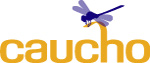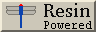EJB-QL SyntaxResin 3.0 Features Installation Configuration Web Applications IOC/AOP Resources JSP Servlets and Filters Portlets Databases Admin (JMX) CMP EJB Amber EJB 3.0 Security XML and XSLT XTP JMS Performance Protocols Third-party Troubleshooting/FAQ User's Guide Reference Tutorials Scrapbook EJBServer EJB Config EJB-QL Syntax Xdoclet tags?n A find or select method argument FROM Specifies the query's schema and variables IS [NOT] NULL Tests for a null value LIMIT For a collection-valued query, limits the number of items returned NOT expr Boolean not expression OFFSET For a collection-valued query, returns items starting from the offset value ORDER BY Sorts the returned values SELECT Specifies the query's values WHERE Restricts the selected values to those matching a boolean expression bean-expr . cmp-field Selects a field value from an entity bean bean-expr . cmr-field Selects relation bean from an entity bean expr + expr Arithmetic expressions expr = expr Comparison expressions expr AND expr Boolean and expression expr OR expr Boolean or expression fun_name(expr, ...) Applies a function to the expression arguments

 ```SELECT expr FROM schema AS var, IN(path) AS var1, ... [WHERE expr] [ORDER BY expr] [OFFSET integer] [LIMIT integer] ```

The WHERE and ORDER BY clauses are optional.

#### SELECT

Specifies the query's values.

 ```SELECT DISTINCT? expr ```

#### FROM

Specifies the query's schema and variables.

 ```FROM schema AS var, IN(path) AS var1, ... ```

#### WHERE

Restricts the selected values to those matching a boolean expression.

 ```WHERE boolean-expr ```

#### ORDER BY

Resin-CMP 1.0

Sorts the returned values. The sorts the results of a query by an expression. Because the database sorts the results, ORDER BY can often be more efficient than sorting in Java in addition to being clearer.

The ORDER BY expression may use a string argument, like ?3. This allows the ORDER BY field to be selected by the argument.

 ```ORDER BY? expr ```

#### OFFSET

Resin-CMP 2.1.1

For a collection-valued query, returns items starting from the offset value. Only integer constants and integer arguments like ?3 are allowed.

OFFSET is generally used in combination with LIMIT to select a slice of a large database query.

Depending on the database, this may require an ORDER BY to make the results consistent. Unless you specify ORDER BY, databases are allowed to return query results in any order. So using OFFSET without ORDER BY name return different results even when called with the same query.

 ```SELECT o FROM items o ORDER BY o.id OFFSET ?1 LIMIT ?2 ```

#### LIMIT

Resin-CMP 2.1.1

For a collection-valued query, limits the number of items returned. Only integer constants and integer arguments like ?3 are allowed.

LIMIT is generally used with OFFSET to handle large database queries which may have multiple return pages.

Depending on the database, LIMIT may require an ORDER BY to make the results consistent. Unless you specify ORDER BY, databases are allowed to return query results in any order. So using OFFSET without ORDER BY name return different results even when called with the same query.

 ```SELECT o FROM items o ORDER BY o.id OFFSET ?1 LIMIT ?2 ```

#### bean-expr . cmp-field

Selects a field value from an entity bean. The type of the expression is the type of the cmp-field.

 ```SELECT c FROM courses c WHERE c.room = 'Leaky Dungeon' ```

#### bean-expr . cmr-field

Selects relation bean from an entity bean. The type of the expression is the bean result. Because the value of the expression is an entity bean, the expression can be used in a further path expression.

 ```SELECT c FROM courses c WHERE c.teacher.name = 'Severus Snape' ```

#### ?n

A find or select method argument. The value of the argument is inserted into the expression. The count is 1-based, so a method with a single argument will use ?1. The type of the expression is the type of the method argument.

 ```SELECT c FROM courses c WHERE c.room = ?1 ```

#### expr + expr

Arithmetic expressions. Adds, subtracts, multiplies or divides two expressions, returning a numeric expression. The sub-expressions must have numeric values.

OperatorMeaning
-Subtract
*Multiply
/Divide

#### expr = expr

Comparison expressions. Compares two expressions, returning a boolean expression. The two expressions for an equality comparison must have compatible types. The expressions for less-than comparison must have numeric values.

OperatorMeaning
=Equals
<>Not equals
<Less-than
<=Less-than or equal to
>Greater-than
>=Greater-than or equal to

 ```SELECT c FROM courses c WHERE c.students < 20 ```

#### IS [NOT] NULL

Tests for a null value. IS NULL can be used both for SQL null values and for null relation values.

The following example shows the case where c.teacher is a relation to a Teacher object. It will select courses with no assigned teacher.

 ```SELECT c FROM courses c WHERE c.teacher IS NULL ```

#### expr AND expr

Boolean and expression. Evaluates to true if both expressions are true. Both expressions must be boolean and the result is a boolean.

#### expr OR expr

Boolean or expression. Evaluates to true if either expression is true. Both expressions must be boolean and the result is a boolean.

#### NOT expr

Boolean not expression. The expression and the result are boolean expressions.

#### fun_name(expr, ...)

Applies a function to the expression arguments. Resin-CMP will allow the functions defined in the JDBC 2.0 spec, but the EJB-QL spec only defines the following functions:

FunctionDescription
CONCAT(string, string)Contatenates two strings
SUBSTRING(string, start, len)Selects a substring
LOCATE(string, start [, start])Finds a substring
LENGTH(string)Returns the string length
ABS(number)Returns the absolute value
SQRT(double)Returns the square root of a number

Additional functions can be added using the query-function tag in the resin-ejb configuration file.

The following functions are known to Resin-CMP:

 ```int abs(int) double abs(double) double acos(double) double sin(double) double atan(double) double cos(double) double cot(double) double degrees(double) double exp(double) double log(double) double log10(double) double radians(double) double sin(double) double sqrt(double) double tan(double) int ceiling(double) int floor(double) int sign(double) double atan2(double, double) double power(double, double) double round(double, double) double truncate(double, double) int mod() int rand() int count(any) double min(double) double max(double) int ascii(String) int length(String) String char(int) String space(int) String concat(String, String) int difference(String, String) String insert(String, int, int, String) String lcase(String) String ltrim(String) String rtrim(String) String ucase(String) String soundex(String) String left(String, int) String repeat(String, int) String right(String, int) String locate(String, int) String locate(String, int, int) String replace() String substring(String, int, int) String database() String user() Date curdate() Date curtime() Date now() String dayname(Date) String monthname(Date) int dayofmonth(Date) int dayofweek(Date) int dayofyear(Date) int hour(Date) int minute(Date) int month(Date) int quarter(Date) int second(Date) int week(Date) int year(Date) Date timestampadd(Date, Date) Date timestampdiff(Date, Date) ```

 Copyright © 1998-2005 Caucho Technology, Inc. All rights reserved. Resin® is a registered trademark, and HardCoretm and Quercustm are trademarks of Caucho Technology, Inc.